# 具有阶段结构和双线性发生率的HIV模型的稳定性分析Stability Analysis of HIV Models with Stage Structure and Bilinear Incidence

DOI: 10.12677/AAM.2019.82019, PDF, HTML, XML, 下载: 510  浏览: 1,688  科研立项经费支持

Abstract: AIDS is one of the most harmful infectious diseases. In this paper, we study a class of HIV trans-mission models with stage structure and bilinear incidence. The spectral radius method is used to calculate the basic regeneration number R0. Furthermore, we prove that the system has a unique disease-free equilibrium E0 when R0<1 while its global asymptotic stability is obtained by the V-function method and the LaSalle invariant principle; and when R0>1, the system adds an en-demic equilibrium E* which is globally asymptotically stable. Numerical simulations are carried out to verify our theoretical results.

1. 引言Figure 1. The clinical process of HIV infection in adults

2. 模型建立及预备结论

1) 人口增加率为常数k，且均为易感者。

2) HIV携带者与易感者有相同的自然死亡率。

$\left\{\begin{array}{l}\stackrel{˙}{S}=k-dS-S\left({\beta }_{1}{I}_{1}+{\beta }_{2}{I}_{2}+{\beta }_{3}{I}_{3}\right),\\ {\stackrel{˙}{I}}_{1}=-d{I}_{1}-{\omega }_{1}{I}_{1}+S\left({\beta }_{1}{I}_{1}+{\beta }_{2}{I}_{2}+{\beta }_{3}{I}_{3}\right),\\ {\stackrel{˙}{I}}_{2}=-d{I}_{2}-{\omega }_{2}{I}_{2}+{\omega }_{1}{I}_{1},\\ {\stackrel{˙}{I}}_{3}=-d{I}_{3}-{\omega }_{3}{I}_{3}+{\omega }_{2}{I}_{2},\\ S\left(0\right)={S}^{0},\text{\hspace{0.17em}}{I}_{1}\left(0\right)={I}_{1}^{0},\text{\hspace{0.17em}}{I}_{2}\left(0\right)={I}_{2}^{0},\text{\hspace{0.17em}}{I}_{3}\left(0\right)={I}_{3}^{0},\end{array}$ (1)

${S}^{0}>0,\text{\hspace{0.17em}}\text{\hspace{0.17em}}{I}_{1}^{0}>0,\text{\hspace{0.17em}}\text{\hspace{0.17em}}{I}_{2}^{0}>0,\text{\hspace{0.17em}}\text{\hspace{0.17em}}{I}_{3}^{0}>0.$ (2)

$\stackrel{˙}{N}=k-dN-{\omega }_{3}{I}_{3}\le k-dN.$

$0\le N\le \frac{k}{d}+N\left(0\right){\text{e}}^{-dt}.$

$\underset{t\to \infty }{\mathrm{lim}\mathrm{sup}}N\left(t\right)\le \frac{k}{d}.$

3. 模型的无病平衡点和基本再生数

${I}_{1}={I}_{2}={I}_{3}=0$ 可得系统(1)的无病平衡点 ${E}^{0}\left(\frac{k}{d},0,0,0\right)$ 。记 ${S}_{0}=\frac{k}{d}$ 。下面我们用再生矩阵的方法   求得基本再生数。

$X=\left({I}_{1},{I}_{2},{I}_{3},S\right)$ 则系统(1)变成 $\stackrel{˙}{X}=\Re \left(x\right)-\aleph \left(x\right)$ ，其中：

$\Re \left(x\right)=\left(\begin{array}{l}S\left({\beta }_{1}{I}_{1}+{\beta }_{2}{I}_{2}+{\beta }_{3}{I}_{3}\right)\\ \text{}0\\ \text{}0\\ \text{}0\end{array}\right),\text{}\aleph \left(X\right)=\left(\begin{array}{l}\text{}\left(d+{\omega }_{1}\right){I}_{1}\\ \text{}\left(d+{\omega }_{2}\right){I}_{2}-{\omega }_{1}{I}_{1}\\ \text{}\left(d+{\omega }_{3}\right){I}_{3}-{\omega }_{2}{I}_{2}\\ S\left({\beta }_{1}{I}_{1}+{\beta }_{2}{I}_{2}+{\beta }_{3}{I}_{3}\right)+dS-k\end{array}\right).$

$\Re \left(x\right),\aleph \left(x\right)$${E}^{0}$ 处的Jacobian矩阵分别为：

$R=\left[\begin{array}{cccc}{S}_{0}{\beta }_{1}& {S}_{0}{\beta }_{2}& {S}_{0}{\beta }_{3}& 0\\ 0& 0& 0& 0\\ 0& 0& 0& 0\\ 0& 0& 0& 0\end{array}\right]，\text{}H=\left[\begin{array}{cccc}d+{\omega }_{1}& 0& 0& 0\\ -{\omega }_{1}& d+{\omega }_{2}& 0& 0\\ 0& -{\omega }_{2}& d+{\omega }_{3}& 0\\ {S}_{0}{\beta }_{1}& {S}_{0}{\beta }_{2}& {S}_{0}{\beta }_{3}& d\end{array}\right].$

${R}_{0}=\rho \left(R{H}^{-1}\right)=\frac{{S}_{0}{\beta }_{1}\left(d+{\omega }_{2}\right)\left(d+{\omega }_{3}\right)+{S}_{0}{\beta }_{2}{\omega }_{1}\left(d+{\omega }_{3}\right)+{S}_{0}{\beta }_{3}{\omega }_{1}{\omega }_{2}}{\left(d+{\omega }_{1}\right)\left(d+{\omega }_{2}\right)\left(d+{\omega }_{3}\right)}.$

$J\left({E}^{0}\right)=\left(\begin{array}{cccc}-d& -{S}_{0}{\beta }_{1}& -{S}_{0}{\beta }_{2}& -{S}_{0}{\beta }_{3}\\ 0& -d-{\omega }_{1}+{S}_{0}{\beta }_{1}& {S}_{0}{\beta }_{2}& {S}_{0}{\beta }_{3}\\ 0& {\omega }_{1}& -d-{\omega }_{2}& 0\\ 0& 0& {\omega }_{2}& -d-{\omega }_{3}\end{array}\right).$

$A=\left(\begin{array}{ccc}-d-{\omega }_{1}+{S}_{0}{\beta }_{1}& {S}_{0}{\beta }_{2}& {S}_{0}{\beta }_{3}\\ {\omega }_{1}& -d-{\omega }_{2}& 0\\ 0& {\omega }_{2}& -d-{\omega }_{3}\end{array}\right).$

$\begin{array}{c}\mathrm{det}\left(A\right)={S}_{0}{\beta }_{3}{\omega }_{1}{\omega }_{2}-\left(d+{\omega }_{3}\right)\left[d\left(d+{\omega }_{3}\right)-{S}_{0}{\beta }_{1}\left(d+{\omega }_{2}\right)+{\omega }_{1}\left(-{S}_{0}{\beta }_{2}+d+{\omega }_{2}\right)\right]\\ =\left(d+{\omega }_{1}\right)\left(d+{\omega }_{2}\right)\left(d+{\omega }_{3}\right)\left[\frac{{S}_{0}{\beta }_{1}\left(d+{\omega }_{2}\right)\left(d+{\omega }_{3}\right)+{S}_{0}{\beta }_{1}{\omega }_{1}\left(d+{\omega }_{3}\right)+{S}_{0}{\beta }_{3}{\omega }_{1}{\omega }_{2}}{\left(d+{\omega }_{1}\right)\left(d+{\omega }_{2}\right)\left(d+{\omega }_{3}\right)}-1\right]\\ =\left(d+{\omega }_{1}\right)\left(d+{\omega }_{2}\right)\left(d+{\omega }_{3}\right)\left({R}_{0}-1\right).\end{array}$

${R}_{0}>1$$\mathrm{det}\left(A\right)>0$ 。此时 $J\left({E}^{0}\right)$ 必有一个正的特征根，因此无病平衡的 ${E}^{0}$ 是不稳定的。下面说明当 ${R}_{0}<1$ 时， ${E}^{0}$ 是局部渐近稳定的。

$Tr\left(A\right)=-3d-{\omega }_{1}-{\omega }_{2}-{\omega }_{3}+{S}_{0}{\beta }_{1}$ .

${A}^{\left[2\right]}=\left(\begin{array}{ccc}-d-{\omega }_{1}+{S}_{0}{\beta }_{1}-d-{\omega }_{2}& 0& -{S}_{0}{\beta }_{3}\\ {\omega }_{2}& -d-{\omega }_{1}+{S}_{0}{\beta }_{1}-d-{\omega }_{3}& 0\\ 0& {\omega }_{1}& -d-{\omega }_{2}-d-{\omega }_{3}\end{array}\right).$

$\begin{array}{c}Tr\left({A}^{\left[2\right]}\right)={S}_{0}{\omega }_{1}\left[-{\beta }_{3}{\omega }_{2}+{\beta }_{2}\left(2d-{S}_{0}{\beta }_{1}+{\omega }_{1}+{\omega }_{2}\right)\right]\\ \text{\hspace{0.17em}}\text{\hspace{0.17em}}-\left(2d-{S}_{0}{\beta }_{1}+{\omega }_{1}+{\omega }_{2}\right)\left(2d-{S}_{0}{\beta }_{1}+{\omega }_{1}+{\omega }_{3}\right)\left(2d+{\omega }_{2}+{\omega }_{3}\right)\end{array}$

${R}_{0}<1$$Tr\left({A}^{\left[2\right]}\right)<0$ 。因此无病平衡点 ${E}^{0}$ 是局部渐近稳定的  。

$V\left(S,{I}_{1},{I}_{2},{I}_{3}\right)=\left(S-{S}_{0}-{S}_{0}ln\frac{S}{{S}_{0}}\right)+{a}_{1}{I}_{1}+{a}_{2}{I}_{2}+{a}_{3}{I}_{3}.$

$\begin{array}{c}\stackrel{˙}{V}=\stackrel{˙}{S}\left(1-\frac{{S}_{0}}{S}\right)+{a}_{1}{\stackrel{˙}{I}}_{1}+{a}_{2}{\stackrel{˙}{I}}_{2}+{a}_{3}{\stackrel{˙}{I}}_{3}=\left(1-\frac{{S}_{0}}{S}\right)\left[k-dS-S\left({\beta }_{1}{I}_{1}+{\beta }_{2}{I}_{2}+{\beta }_{3}{I}_{3}\right)\right]\\ \text{\hspace{0.17em}}\text{\hspace{0.17em}}+{a}_{1}\left[-d{I}_{1}-{\omega }_{1}{I}_{1}+S\left({\beta }_{1}{I}_{1}+{\beta }_{2}{I}_{2}+{\beta }_{3}{I}_{3}\right)\right]\\ \text{\hspace{0.17em}}\text{\hspace{0.17em}}+{a}_{2}\left(-d{I}_{2}-{\omega }_{2}{I}_{2}+{\omega }_{1}{I}_{1}\right)+{a}_{3}\left(-d{I}_{3}-{\omega }_{3}{I}_{3}+{\omega }_{2}{I}_{2}\right)\end{array}$

$\begin{array}{c}=-dS{\left(1-\frac{{S}_{0}}{S}\right)}^{2}-S\left({\beta }_{1}{I}_{1}+{\beta }_{2}{I}_{2}+{\beta }_{3}{I}_{3}\right)+{S}_{0}\left({\beta }_{1}{I}_{1}+{\beta }_{2}{I}_{2}+{\beta }_{3}{I}_{3}\right)\\ \text{\hspace{0.17em}}\text{\hspace{0.17em}}+{a}_{1}\left[-d{I}_{1}-{\omega }_{1}{I}_{1}+S\left({\beta }_{1}{I}_{1}+{\beta }_{2}{I}_{2}+{\beta }_{3}{I}_{3}\right)\right]\\ \text{\hspace{0.17em}}\text{\hspace{0.17em}}+{a}_{2}\left(-d{I}_{2}-{\omega }_{2}{I}_{2}+{\omega }_{1}{I}_{1}\right)+{a}_{3}\left(-d{I}_{3}-{\omega }_{3}{I}_{3}+{\omega }_{2}{I}_{2}\right)\end{array}$

${a}_{1}=1,\text{\hspace{0.17em}}{a}_{2}=\frac{{S}_{0}{\beta }_{2}\left(d+{\omega }_{3}\right)+{\omega }_{2}{S}_{0}{\beta }_{3}}{\left(d+{\omega }_{2}\right)\left(d+{\omega }_{3}\right)},\text{\hspace{0.17em}}{a}_{3}=\frac{{S}_{0}{\beta }_{3}}{d+{\omega }_{3}}$ ，则上式右端可变成：

$\begin{array}{c}\stackrel{˙}{V}=-dS{\left(1-\frac{{S}_{0}}{S}\right)}^{2}+\left[{S}_{0}{\beta }_{1}-\left(d+{\omega }_{1}\right)+{a}_{2}{\omega }_{1}\right]{I}_{1}=-dS{\left(1-\frac{{S}_{0}}{S}\right)}^{2}-\left(d+{\omega }_{1}\right)\left[1-\frac{{S}_{0}{\beta }_{1}+{a}_{2}{\omega }_{1}}{d+{\omega }_{1}}\right]{I}_{1}\\ =-dS{\left(1-\frac{{S}_{0}}{S}\right)}^{2}-\left(d+{\omega }_{1}\right)\left(1-{R}_{0}\right){I}_{1}.\end{array}$

4. 地方病平衡点的存在性和渐近稳定性

$\left\{\begin{array}{l}k-dS-S\left({\beta }_{1}{I}_{1}+{\beta }_{2}{I}_{2}+{\beta }_{3}{I}_{3}\right)=0\\ -d{I}_{1}-{\omega }_{1}{I}_{1}+S\left({\beta }_{1}{I}_{1}+{\beta }_{2}{I}_{2}+{\beta }_{3}{I}_{3}\right)=0\\ -d{I}_{2}-{\omega }_{2}{I}_{2}+{\omega }_{1}{I}_{1}=0\\ -d{I}_{3}-{\omega }_{3}{I}_{3}+{\omega }_{2}{I}_{2}=0\end{array}$

${S}^{*}=\frac{{S}_{0}}{{R}_{0}},\text{\hspace{0.17em}}\text{\hspace{0.17em}}{I}_{2}^{*}=\frac{d{S}_{0}{\omega }_{1}\left({R}_{0}-1\right)}{{R}_{0}\left(d+{\omega }_{1}\right)\left(d+{\omega }_{2}\right)},\text{\hspace{0.17em}}\text{\hspace{0.17em}}{I}_{1}^{*}=\frac{\left(d+{\omega }_{2}\right){I}_{2}^{*}}{{\omega }_{1}},\text{\hspace{0.17em}}\text{\hspace{0.17em}}{I}_{3}^{*}=\frac{{\omega }_{2}{I}_{2}^{*}}{d+{\omega }_{3}}.$

$\mathrm{min}\left\{\underset{t\to +\infty }{\mathrm{lim}\mathrm{inf}}S\left(t\right),\underset{t\to +\infty }{\mathrm{lim}\mathrm{inf}}{I}_{1}\left(t\right),\underset{t\to +\infty }{\mathrm{lim}\mathrm{inf}}{I}_{2}\left(t\right),\underset{t\to +\infty }{\mathrm{lim}\mathrm{inf}}{I}_{3}\left(t\right)\right\}>C.$

$V\left(S,{I}_{1},{I}_{2},{I}_{3}\right)=\left(S-{S}^{*}-{S}^{*}ln\frac{S}{{S}^{*}}\right)+{a}_{1}\left({I}_{1}-{I}_{1}^{*}-{I}_{1}^{*}\mathrm{ln}\frac{{I}_{1}}{{I}_{1}^{*}}\right)\text{\hspace{0.17em}}\text{\hspace{0.17em}}+{a}_{2}\left({I}_{2}-{I}_{2}^{*}-{I}_{2}^{*}\mathrm{ln}\frac{{I}_{2}}{{I}_{2}^{*}}\right)+{a}_{3}\left({I}_{3}-{I}_{3}^{*}-{I}_{3}^{*}\mathrm{ln}\frac{{I}_{3}}{{I}_{3}^{*}}\right).$

$\begin{array}{c}\stackrel{˙}{V}=\left(1-\frac{{S}^{*}}{S}\right)\stackrel{˙}{S}+{a}_{1}\left(1-\frac{{I}_{1}^{*}}{{I}_{1}}\right){\stackrel{˙}{I}}_{1}+{a}_{2}\left(1-\frac{{I}_{2}^{*}}{{I}_{2}}\right){\stackrel{˙}{I}}_{2}+{a}_{3}\left(1-\frac{{I}_{3}^{*}}{{I}_{3}}\right){\stackrel{˙}{I}}_{3}\\ =\left(1-\frac{{S}^{*}}{S}\right)\left[k-dS-S\left({\beta }_{1}{I}_{1}+{\beta }_{2}{I}_{2}+{\beta }_{3}{I}_{3}\right)\right]\\ \text{\hspace{0.17em}}\text{\hspace{0.17em}}+{a}_{1}\left(1-\frac{{I}_{1}^{*}}{{I}_{1}}\right)\left[-d{I}_{1}-{\omega }_{1}{I}_{1}+S\left({\beta }_{1}{I}_{1}+{\beta }_{2}{I}_{2}+{\beta }_{3}{I}_{3}\right)\right]\\ \text{\hspace{0.17em}}\text{\hspace{0.17em}}+{a}_{2}\left(1-\frac{{I}_{2}^{*}}{{I}_{2}}\right)\left(-d{I}_{2}-{\omega }_{2}{I}_{2}+{\omega }_{1}{I}_{1}\right)+{a}_{3}\left(1-\frac{{I}_{3}^{*}}{{I}_{3}}\right)\left(-d{I}_{3}-{\omega }_{3}{I}_{3}+{\omega }_{2}{I}_{2}\right)\end{array}$ (3)

$\begin{array}{l}=\left(k+d{S}^{*}+{a}_{1}d{I}_{1}^{*}+{a}_{1}{\omega }_{1}{I}_{1}^{*}+{a}_{2}d{I}_{2}^{*}+{a}_{2}{\omega }_{2}{I}_{2}^{*}+{a}_{3}d{I}_{3}^{*}+{a}_{3}{\omega }_{3}{I}_{3}^{*}\right)+\left(-d-{a}_{1}{\beta }_{1}{I}_{1}^{*}\right)S\\ \text{\hspace{0.17em}}\text{\hspace{0.17em}}\text{ }\text{ }+\left({a}_{1}-1\right)S\left({\beta }_{1}{I}_{1}+{\beta }_{2}{I}_{2}+{\beta }_{3}{I}_{3}\right)+\left({S}^{*}{\beta }_{1}-{a}_{1}d-{a}_{1}{\omega }_{1}+{a}_{2}{\omega }_{1}\right){I}_{1}\\ \text{\hspace{0.17em}}\text{\hspace{0.17em}}\text{ }\text{ }+\left({S}^{*}{\beta }_{2}-{a}_{2}d-{a}_{2}{\omega }_{2}+{a}_{3}{\omega }_{2}\right){I}_{2}+\left({S}^{*}{\beta }_{3}-{a}_{3}d-{a}_{3}{\omega }_{3}\right){I}_{3}\\ \text{\hspace{0.17em}}\text{\hspace{0.17em}}\text{ }\text{ }+\left(-k\frac{{S}^{*}}{S}-{a}_{1}{\beta }_{2}{I}_{1}^{*}\frac{S{I}_{2}}{{I}_{1}}-{a}_{1}{\beta }_{3}{I}_{1}^{*}\frac{S{I}_{3}}{{I}_{1}}-{a}_{2}{\omega }_{1}{I}_{2}^{*}\frac{{I}_{1}}{{I}_{2}}-{a}_{3}{\omega }_{2}{I}_{3}^{*}\frac{{I}_{2}}{{I}_{3}}\right)\end{array}$

$\begin{array}{l}c=k+d{S}^{*}+{a}_{1}d{I}_{1}^{*}+{a}_{1}{\omega }_{1}{I}_{1}^{*}+{a}_{2}d{I}_{2}^{*}+{a}_{2}{\omega }_{2}{I}_{2}^{*}+{a}_{3}d{I}_{3}^{*}+{a}_{3}{\omega }_{3}{I}_{3}^{*},\\ w=\frac{S}{{S}^{*}},\text{}x=\frac{{I}_{1}}{{I}_{1}^{*}},\text{}y=\frac{{I}_{2}}{{I}_{2}^{*}},\text{}z=\frac{{I}_{3}}{{I}_{3}^{*}}.\end{array}$

${b}_{1}\left(2-w-\frac{1}{w}\right)+{b}_{2}\left(4-\frac{1}{w}-\frac{wz}{x}-\frac{y}{z}-\frac{x}{y}\right)+{b}_{3}\left(3-\frac{1}{w}-\frac{wy}{x}-\frac{x}{y}\right),$

$\begin{array}{ccc}\left\{\begin{array}{l}2{b}_{1}+4{b}_{2}+3{b}_{3}=c\\ {b}_{1}=\left(d+{a}_{1}{I}_{1}^{*}{\beta }_{1}\right){S}^{*}\\ {a}_{1}-1=0\\ {b}_{1}+{b}_{2}+{b}_{3}=k\\ -{a}_{2}\left(d+{\omega }_{2}\right)+{a}_{2}{\omega }_{2}+{S}^{*}{\beta }_{2}=0\\ -{a}_{3}\left(d+{\omega }_{3}\right)+{S}^{*}{\beta }_{3}=0\\ {b}_{3}={S}^{*}{\beta }_{2}{I}_{2}^{*}\\ {b}_{2}={S}^{*}{\beta }_{3}{I}_{3}^{*}={a}_{3}{\omega }_{2}{I}_{2}^{*}\\ {b}_{2}+{b}_{3}={a}_{2}{\omega }_{1}{I}_{1}^{*}\end{array}& ⇔& \left\{\begin{array}{l}{b}_{1}=\left(d+{a}_{1}{I}_{1}^{*}{\beta }_{1}\right){S}^{*}>0\\ {b}_{2}={S}^{*}{\beta }_{3}{I}_{3}^{*}>0\\ {b}_{3}={S}^{*}{\beta }_{2}{I}_{2}^{*}>0\\ {a}_{1}=1\\ {a}_{2}=\frac{{S}^{*}{\beta }_{3}{\omega }_{2}+{S}^{*}{\beta }_{2}\left(d+{\omega }_{3}\right)}{\left(d+{\omega }_{2}\right)\left(d+{\omega }_{3}\right)}\\ {a}_{3}=\frac{{S}^{*}{\beta }_{3}}{d+{\omega }_{3}}\end{array}\end{array}$

$\stackrel{˙}{V}={b}_{1}\left(2-w-\frac{1}{w}\right)+{b}_{2}\left(4-\frac{1}{w}-\frac{wz}{x}-\frac{y}{z}-\frac{x}{y}\right)+{b}_{3}\left(3-\frac{1}{w}-\frac{wy}{x}-\frac{x}{y}\right)\le 0,$

$\stackrel{˙}{V}=0$ 当且仅当 $w=\frac{1}{w}=\frac{wz}{x}=\frac{y}{z}=\frac{x}{y}=\frac{wy}{x}=1$ ，即 $\left\{\stackrel{˙}{V}=0\right\}=\left\{{E}^{*}\right\}$ 。由LaSalle不变原理知地方病平衡点 ${E}^{\text{*}}$$\stackrel{\circ }{\Omega }$ 上是渐近稳定的。

5. 数值模拟

${S}^{0}=10000,\text{}{I}_{1}^{0}=15,\text{}{I}_{2}^{0}=50,\text{}{I}_{3}^{0}=50.$

1) 当 ${R}_{0}<1$ 时的情形

$\begin{array}{l}k=10,\text{\hspace{0.17em}}\text{\hspace{0.17em}}d=0.01,\text{\hspace{0.17em}}\text{\hspace{0.17em}}{\omega }_{1}=\frac{1}{3},\text{\hspace{0.17em}}\text{\hspace{0.17em}}{\omega }_{2}=\frac{1}{96},\\ {\omega }_{3}=\frac{1}{13},\text{\hspace{0.17em}}\text{\hspace{0.17em}}{\beta }_{1}=2×{10}^{-4},\text{\hspace{0.17em}}\text{\hspace{0.17em}}{\beta }_{2}=8×{10}^{-6},\text{\hspace{0.17em}}\text{\hspace{0.17em}}{\beta }_{3}=7.6×{10}^{-5}.\end{array}$ (4)

$S\left(20000\right)=999.9983,\text{\hspace{0.17em}}\text{\hspace{0.17em}}{I}_{1}\left(20000\right)=4.5139×{10}^{-6},\text{\hspace{0.17em}}{I}_{2}\left(20000\right)=7.4608×{10}^{-4},\text{\hspace{0.17em}}\text{\hspace{0.17em}}{I}_{3}\left(20000\right)=9.1416×{10}^{-5}.$

ii) 当 ${R}_{0}>1$ 时的情形

$\begin{array}{l}k=15,\text{\hspace{0.17em}}\text{\hspace{0.17em}}d=0.01,\text{\hspace{0.17em}}\text{\hspace{0.17em}}{\omega }_{1}=\frac{1}{3},\text{\hspace{0.17em}}\text{\hspace{0.17em}}{\omega }_{2}=\frac{1}{96},\\ {\omega }_{3}=\frac{1}{13},\text{\hspace{0.17em}}\text{\hspace{0.17em}}{\beta }_{1}=2×{10}^{-4},\text{\hspace{0.17em}}\text{\hspace{0.17em}}{\beta }_{2}=8×{10}^{-6},\text{\hspace{0.17em}}\text{\hspace{0.17em}}{\beta }_{3}=7.6×{10}^{-5}.\end{array}$ (5)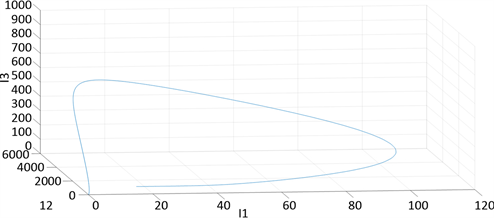Figure 2. Three-dimensional projection of phase portrait of system (1) with ${S}^{0}=10000$, ${I}_{1}^{0}=15$, ${I}_{2}^{0}=50$, ${I}_{3}^{0}=50$, and the parameter values are shown in (4)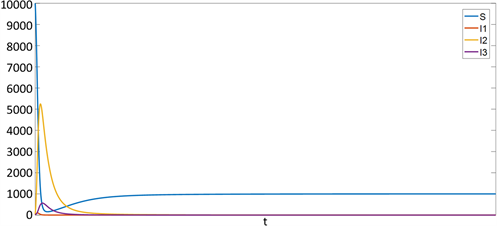Figure 3. Images of $S,{I}_{1},{I}_{2},{I}_{3}$ of the solution of system (1) with ${S}^{0}=10000$, ${I}_{1}^{0}=15$, ${I}_{2}^{0}=50$, ${I}_{3}^{0}=50$ and the parameter values are shown in(4)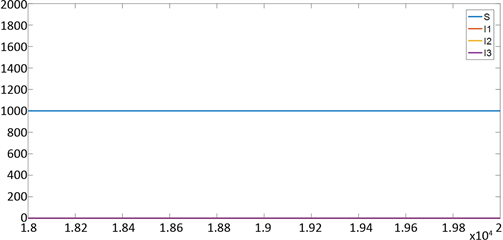Figure 4. Images of $S,{I}_{1},{I}_{2},{I}_{3}$ of the solution of system (1) with ${S}^{0}=10000$, ${I}_{1}^{0}=15$, ${I}_{2}^{0}=50$, ${I}_{3}^{0}=50$ and the parameter values are shown in (4), time of integration: [18000, 20000]Figure 5. Three-dimensional projection of phase portrait of system (1) with ${S}^{0}=10000$, ${I}_{1}^{0}=15$, ${I}_{2}^{0}=50$, ${I}_{3}^{0}=50$, and the parameter values are shown in (5)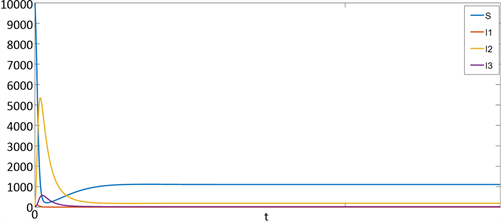Figure 6. Images of $S,{I}_{1},{I}_{2},{I}_{3}$ of the solution of system (1) with ${S}^{0}=10000$, ${I}_{1}^{0}=15$, ${I}_{2}^{0}=50$, ${I}_{3}^{0}=50$ and the parameter values are shown in (5)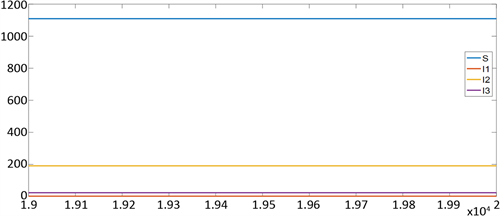Figure 7. Images of $S,{I}_{1},{I}_{2},{I}_{3}$ of the solution of system (1) with ${S}^{0}=10000$, ${I}_{1}^{0}=15$, ${I}_{2}^{0}=50$, ${I}_{3}^{0}=50$ and the parameter values are shown in (5), time of integration : [19000,20000]

${E}^{*}\left(1109.15,1.29849,190.799,22.8649\right).$

$\begin{array}{l}S\left(20000\right)=1.1092×{10}^{3},\text{\hspace{0.17em}}\text{\hspace{0.17em}}{I}_{1}\left(20000\right)=1.2983,\\ {I}_{2}\left(20000\right)=190.7990,\text{\hspace{0.17em}}\text{\hspace{0.17em}}{I}_{3}\left(20000\right)=22.8649.\end{array}$

6. 结论

  马知恩, 周义仓. 传染病动力学的数学建模与研究[M]. 北京: 科学出版社, 2004.  陈雪玲. 一类具有性传播的艾滋病模型的研究[D]: [硕士学位论文]. 乌鲁木齐: 新疆大学, 2017.  买买提江•买斯塔洪. 具有人口迁移的艾滋病模型的稳定性分析及最优控制[D]: [硕士学位论文]. 乌鲁木齐: 新疆大学, 2017.  Mushayabasa, S. (2014) On the role of HIV/AIDS Support Groups on Combating New Infections. HIV & AIDS Review, 13, 109-119. https://doi.org/10.1016/j.hivar.2014.07.002  范新华, 陈荣军. 数学建模[M]. 南京: 南京大学出版社, 2018: 89-94.  梁国业, 廖建平. 数学建模[M]. 北京: 冶金工业出版社, 2004: 256-263.  Lamichhane, S. and Chen, Y.M. (2015) Global Asymptotic Stability of a Compartmental Model for a Pandemic. Journal of the Egyptian Mathematical Society, 23, 251-255. https://doi.org/10.1016/j.joems.2014.04.001  Van den Driessche, P. and Watmough, J. (2002) Reproduction Numbers and Sub-Threshold Endemic Equilibria for Compartmental Models of Disease Transmission. Mathematical Biosciences, 180, 29-48. https://doi.org/10.1016/S0025-5564(02)00108-6  Huo, H.F. and Feng, L.X. (2013) Global Stability for an HIV/AIDS Epidemic Model with Different Latent Stages and Treatment. Applied Mathematical Modelling, 37, 1480-1489. https://doi.org/10.1016/j.apm.2012.04.013  Li, M.Y., Muldowney, J.S. and On, R.A. (1995) Smith’s Autonomous Convergence Theorem. Rocky Mountain Journal of Mathematics, 25, 365-379. https://doi.org/10.1216/rmjm/1181072289  Mccluskey, C.C. and Driessche, P.V.D. (2004) Global Analysis of Two Tuberculosis Models. Journal of Dynamics & Differential Equations, 16, 139-166. https://doi.org/10.1023/B:JODY.0000041283.66784.3e